Microsoft Excel

Microsoft Excel has a number of functions that allow you to complete tasks without the use of a calculator or additional effort. If you're not used to working with formulas, it can be intimidating. To get you started, we've put together a list of 12 basic but important Excel functions.

# SUM Function

Adding numbers is one of the most basic things you can do with them. You can add numbers to cells in Excel using the SUM function. The format is SUM(value1, value2,...), with value1 being required and value2 being optional. You can use a number, a cell reference, or a cell range for each argument.

Suppose, To add the numbers in cells A1 through A10, type the following and hit Enter:
=SUM(A1:A10)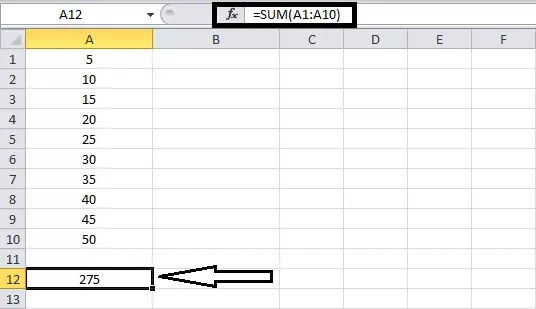SUM Function

# AVERAGE Function

Another frequent mathematical function is to average a bunch of numbers. The AVERAGE function in Excel has the same syntax as the SUM function, AVERAGE(value1, value2,...), with value1 being necessary and value2 being optional. For the arguments, you can use cell references or ranges.

Enter the following formula and click Enter to average the integers in cells A1 through A10.
=AVERAGE(A1:A10)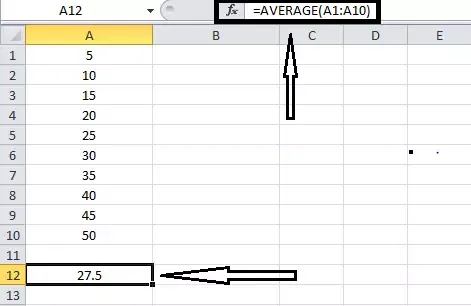Average Function

# MIN & MAX Function

The MIN and MAX functions are used to find the least and maximum values in a range of cells. The syntax for these functions is the same as for the others: MIN(value1, value2,...) and MAX(value1, value2..) (value1, value2,...), with value1 being necessary and value2 being optional.

Enter the following, substituting the cell references with your own, to determine the minimum, lowest value in a collection of cells. Then type for Minimum Value
=MIN(B1:B10)

And type for Maximum Value
=MAX(B1:B10)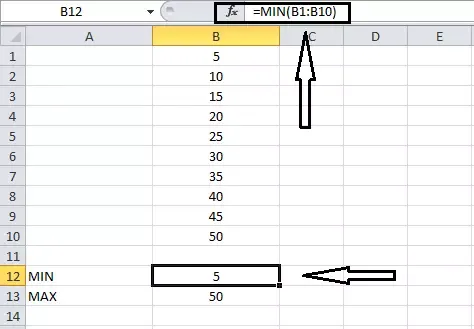MIN & MAX Function

# MEDIAN Function

Instead of using the lowest or highest value, you may utilize the midpoint.  MEDIAN and MEDIAN have the same syntax (value1, value2,...), with the first parameter obligatory and the second optional, as you might expect.

Enter the following and hit Enter for the middle value in a range of cells:
=MEDIAN(B1:B10)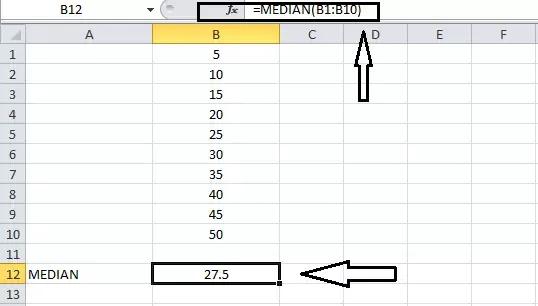MEDIAN Function

# COUNT Function

You might want to count the number of cells in a range that contains numbers. For this, you'd utilize the COUNT function. COUNT(value1, value2,...) has the same syntax as the previous two functions, with the first argument being necessary and the second being optional.

Enter the following and press Enter to count the number of cells that contain numbers in the range B1 through B10:
=COUNT(B1:B10)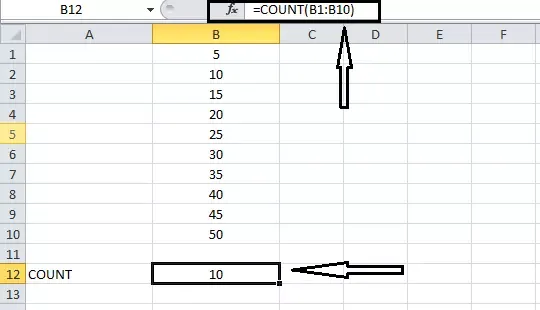Count Function

# NOW Function

Use the NOW function in Excel to display the current date and time anytime you open your spreadsheet. Because the function has no mandatory arguments, the syntax is NOW(). However, by adding or removing objects, you can change the current date and time.

Enter the following and press Enter to return to the current date and time:
=NOW()

Enter this formula and press Enter to return the date and time five days in the future from the current date and time:
=NOW()+5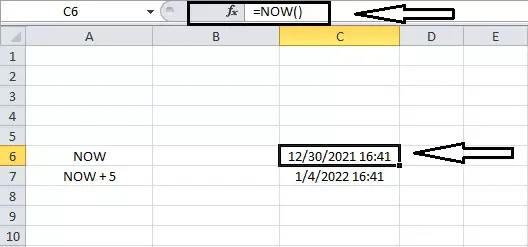NOW Function

# ROUND Function

Use the ROUND function in Excel if you have decimal numbers in your sheet that you wish to round up or down. Both parameters are necessary for the function ROUND(value1, digits) idiom. Use the number you want to round for value1. To round a number with digits, use the number of decimal places.

Enter the following and press Enter to round the figure 3.25 up to one decimal place:
=ROUND(3.25,1)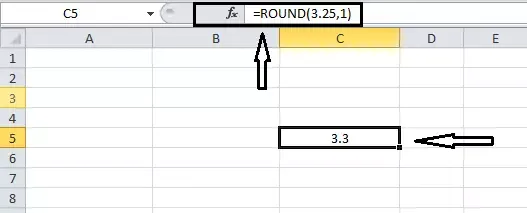ROUND Function

# TRUNC Function

You might prefer to truncate a number rather than round it. Use the TRUNC function to remove a fraction from an integer.

The syntax is TRUNC(value1, digits), with value1 being required and digits being optional. The default value is zero if you don't enter the digits.

Type the following into the box and press Enter to truncate the number 8.2:
=TRUNC(8.2)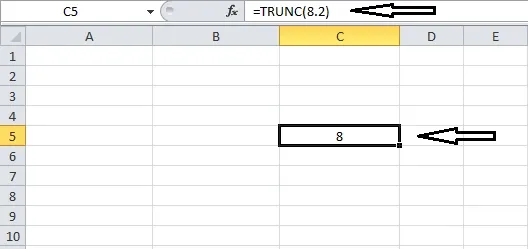TRUNC Function

# PRODUCT Function

The PRODUCT function is more efficient than the multiplication sign (*) in a calculation when multiplying a large number of cells.

The syntax of the PRODUCT function is PRODUCT(value1, value2,...), with value1 being required and value2 being optional. Use value1 for the first cell range and value2 for the second cell range if necessary.

To find the product of cells B4 through B11, type the following and press Enter:
=PRODUCT(B4:B11)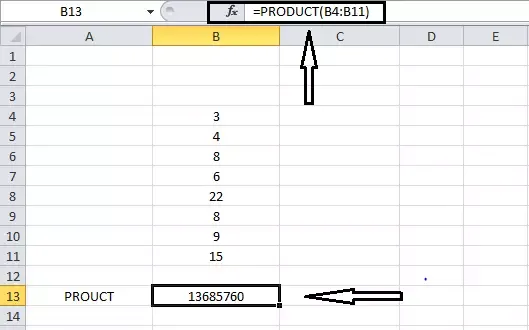PRODUCT Function

# ROWS & COLUMNS Function

The COLUMN and ROW functions in Excel can be used to return a cell's position number. These routines can be used to input a series of reference numbers or row numbers in your spreadsheet. Where the argument is not necessary, the syntax is COLUMN(reference) and ROW(reference). If you don't provide an argument, the formula returns the reference to the formula's cell.

Because C2 is in the second row, the outcome of entering the following formula into cell C2 will be 2.
=ROW()

However, if you enter the following formula with an argument, you'll get the cell's reference number.
=ROW(C5)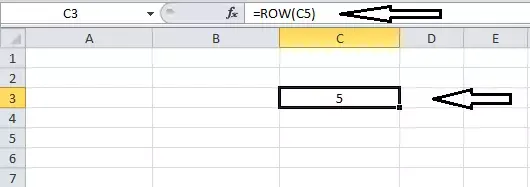ROWS & COLUMNS Function

# TRIM Function

When you paste or import data, it frequently contains additional spaces. White space is removed using the TRIM function. TRIM(reference) is the syntax, with the argument containing the cell reference containing the data.

Enter the following and press Enter to eliminate excess spaces from cell A3:
=TRIM(A3)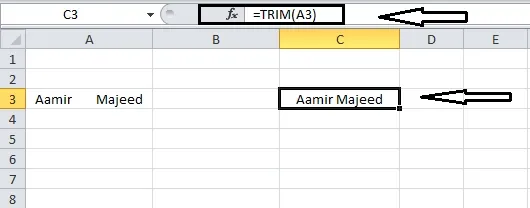TRIM Function

# LEN Function

You might need to figure out how many characters are in a string of text. You'd use Excel's LEN function in this scenario. LEN(reference) is the syntax, with the argument indicating the cell reference containing the text.

Enter the following formula and press Enter to find the number of characters in cell A3:
=LEN(A3)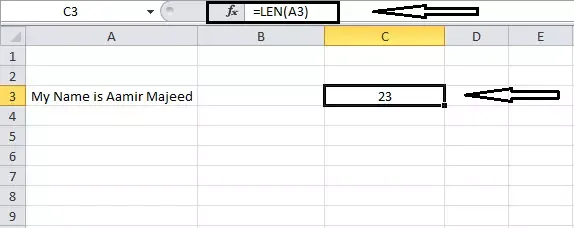LEN Function